# Pictorial circuit diagrams

## Electricity and Electronics

• #### Question 1

Draw connecting wires between the 4-20 mA {\it self-powered} (4-wire) level transmitter, the 24 VDC power supply, and Analog input \#1’’ of the Honeywell UDC2500 controller so that the controller registers the measured level as its process variable (PV). Assume the 4-wire transmitter’s analog output is the {\it sourcing} type: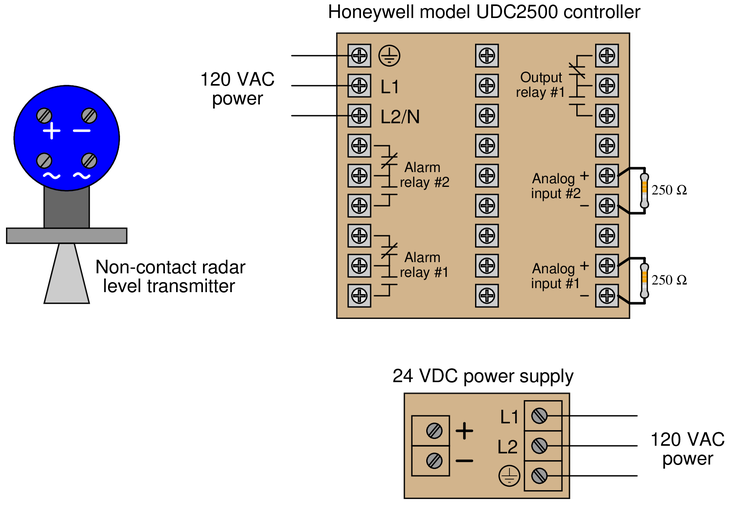• #### Question 2

Sketch connecting wires such that the relay will energize when the normally-open (NO) pushbutton switch is pressed. Be sure to wire the relay in such a way that voltage will appear in the polarities shown by the (+) and ($-$) marks: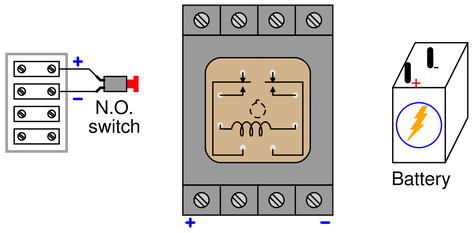• #### Question 3

Sketch connecting wires such that the relay will energize and turn on the lamp when the normally-open (NO) pushbutton switch is pressed. Use the following schematic diagram as a guide: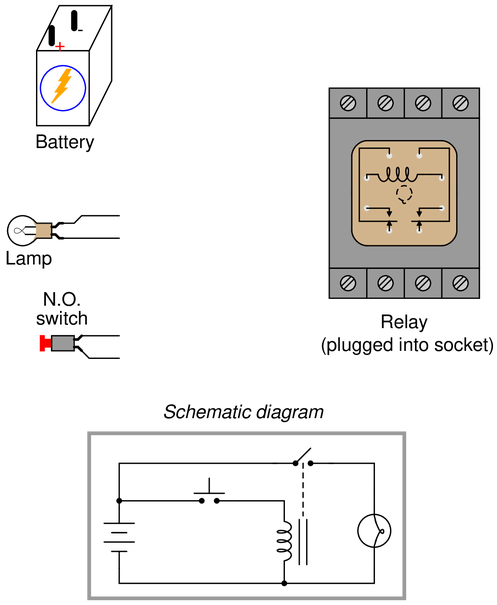Note how the relay coil and lamp are separate (parallel) branches in this circuit. The pushbutton switch {\it only} carries coil current, while the relay’s switch contact {\it only} carries lamp current.

{\bullet} Suppose the battery is rated at 12 volts, the lamp has a hot’’ filament resistance of 3.2 ohms, and the relay coil has a wire resistance of 240 ohms. Calculate the amount of current carried by the switch when it is pressed.

• #### Question 4

Sketch connecting wires such that the relay will energize and turn on the lamp when the normally-open (NO) pushbutton switch is pressed. Be sure to wire the relay in such a way that current (conventional flow) follows the directions indicated by the arrows, and that the switch only carries relay coil current (no lamp current in addition to coil current):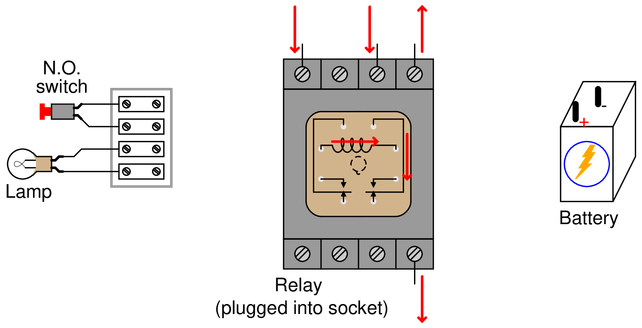• #### Question 5

Sketch connecting wires such that the relay will energize and turn the lamp {\it off} when the normally-open (NO) pushbutton switch is pressed (i.e. the lamp should be {\it on} when the pushbutton switch is not being pressed). Be sure to wire the relay in such a way that current (conventional flow) follows the directions indicated by the arrows, and that the switch only carries relay coil current (no lamp current in addition to coil current):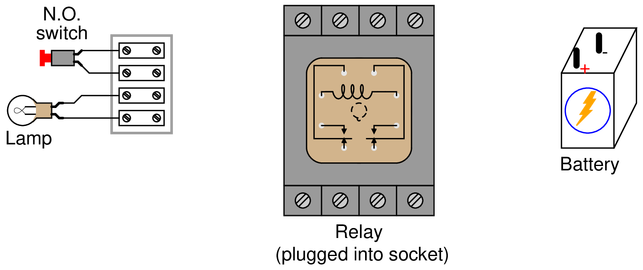• #### Question 6

Sketch connecting wires such that the relay will select one of two different thermocouples to send millivoltage signals to a temperature indicator. Use the following schematic diagram as a guide: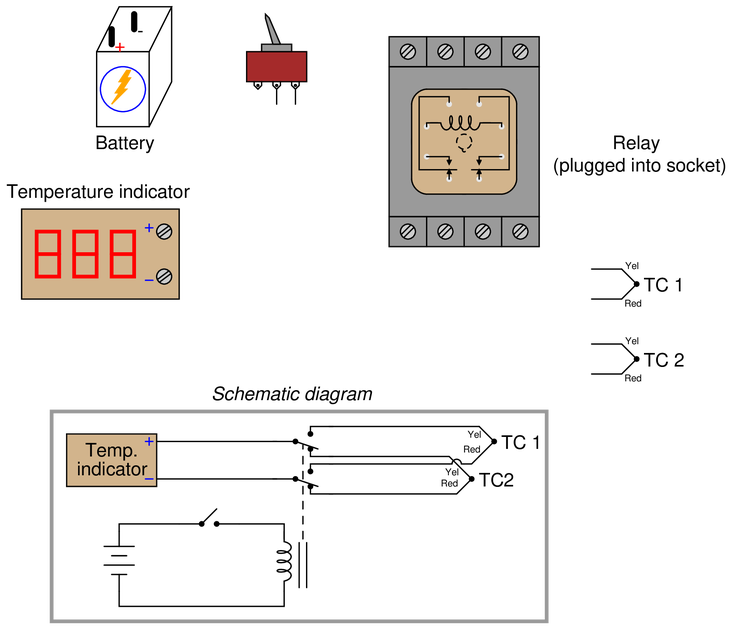• #### Question 7

Draw connecting wires that will create a {\it series} circuit, such that the loop-powered pressure transmitter will drive the ammeter to indicate pressure: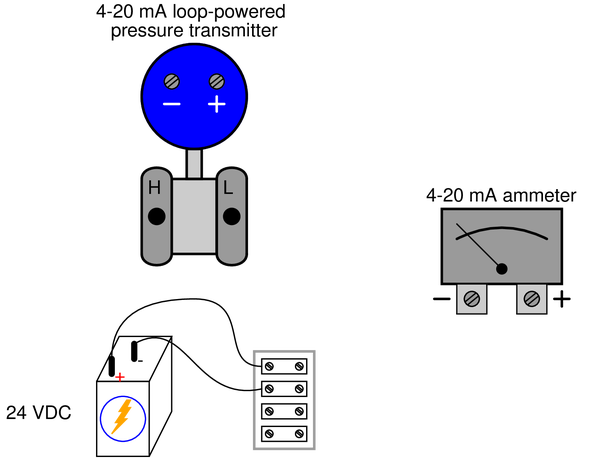• #### Question 8

Draw connecting wires that will create a {\it series} circuit, such that the loop-powered pressure transmitter will drive both ammeters to indicate pressure: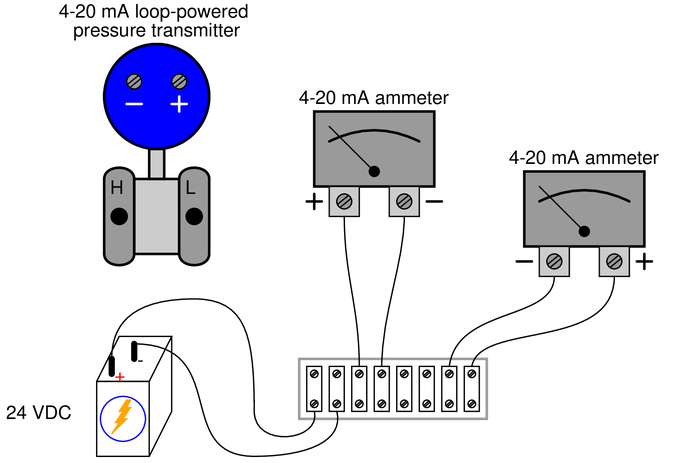• #### Question 9

Some models of the MicroLogix’’ series of programmable logic controller (PLC) manufactured by Allen-Bradley come equipped with analog inputs, designed to receive either voltage or current signals from analog sensors. Examine the internal resistances of the analog inputs (IA/0, IA/1, IA/2, and IA/3) to determine which are designed to input voltage signals and which are designed to input current signals.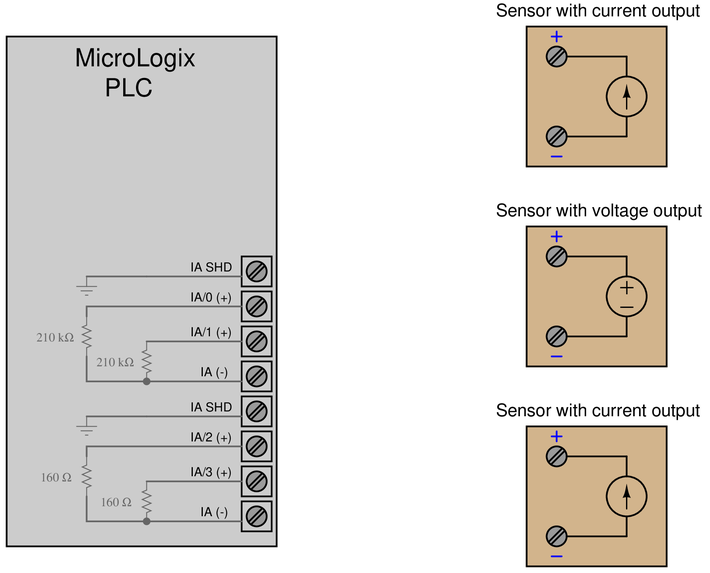Hint: think in terms of the input resistances of {\it voltmeters} and {\it ammeters}. Which of these test instrument types are known for having very large input resistance values? Which of these test instrument types are known for having very small input resistance values?

Assuming the three sensors shown all have internal power sources (no need for an external DC power supply to make them output their respective signals), draw connecting wires between these sensors and the appropriate inputs on the PLC.

• #### Question 10

Draw connecting wires between the 4-20 mA loop-powered pressure transmitter, the 24 VDC power supply, and the PV input’’ of the Honeywell controller so that the controller registers the measured pressure as its process variable (PV):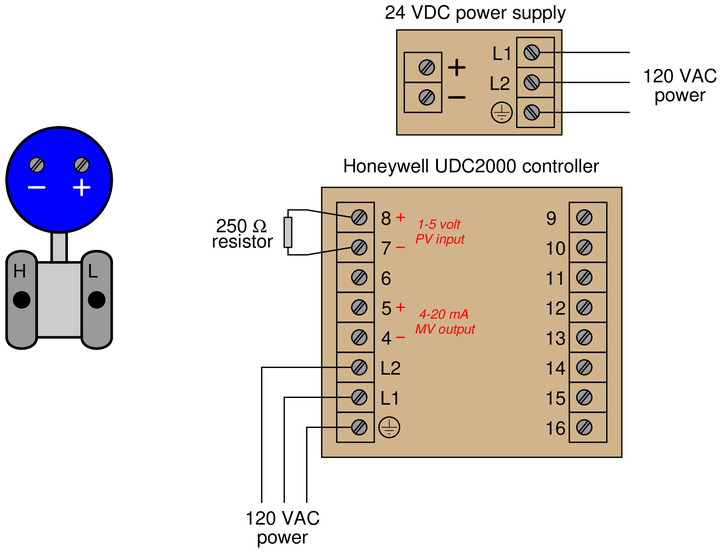• #### Question 11

Draw connecting wires between the 4-20 mA loop-powered pressure transmitter, the 24 VDC power supply, and Analog input \#1’’ of the Honeywell UDC2500 controller so that the controller registers the measured pressure as its process variable (PV):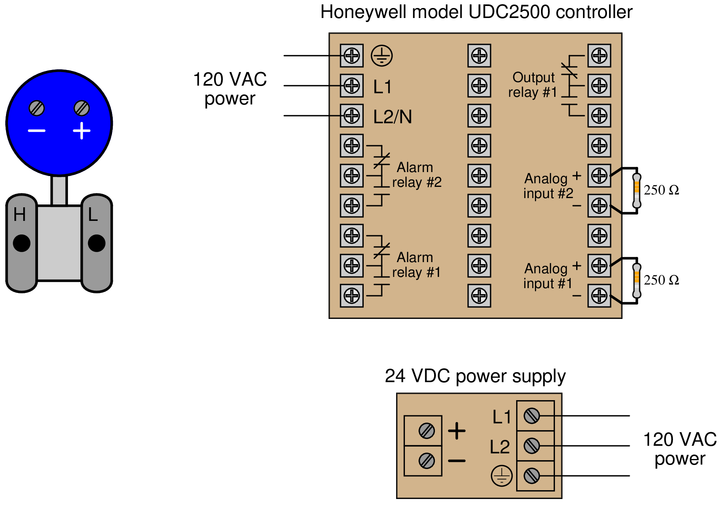• #### Question 12

Sketch connecting wires such that the relay will energize when the normally-open (NO) pushbutton switch is pressed. Be sure to wire the relay in such a way that current (conventional flow) follows the directions indicated by the arrows: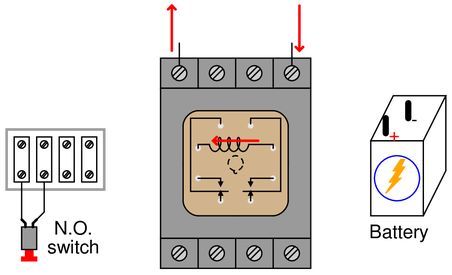• #### Question 13

Sketch connecting wires to allow this data acquisition unit (DAQ) to sense the voltage produced by the solar cell, on input channel \#2: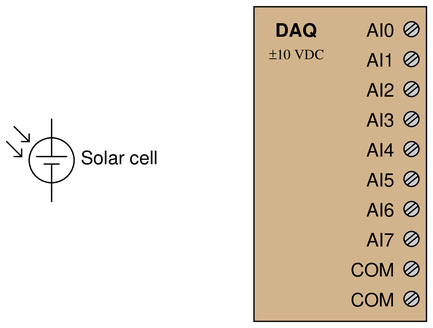Your circuit should be wired in such a way that greater light intensity falling on the cell produces a more {\it positive} signal measured by the DAQ.

• #### Question 14

Sketch connecting wires so that this DAQ unit will register an increasing positive voltage on channel 2 as the potentiometer shaft is turned {\it clockwise}: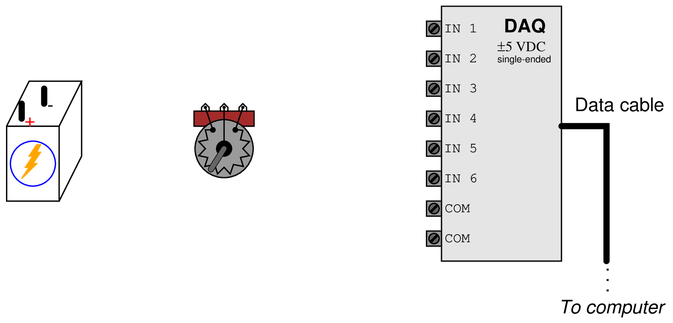• #### Question 15

Sketch connecting wires so that this DAQ unit will register an increasing positive voltage on channel 5 as the potentiometer wiper moves to the {\it left}: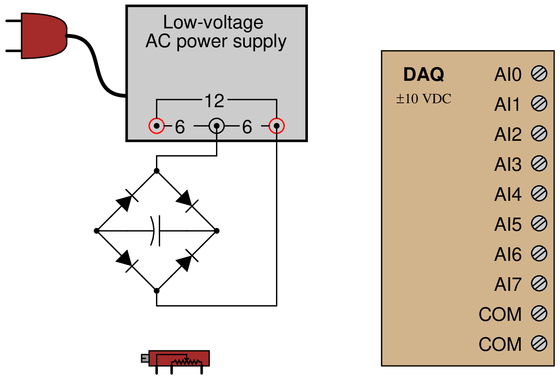• #### Question 16

Sketch connecting wires so that this DAQ unit will register an increasing negative voltage on channel 1 as the potentiometer shaft is turned {\it clockwise}: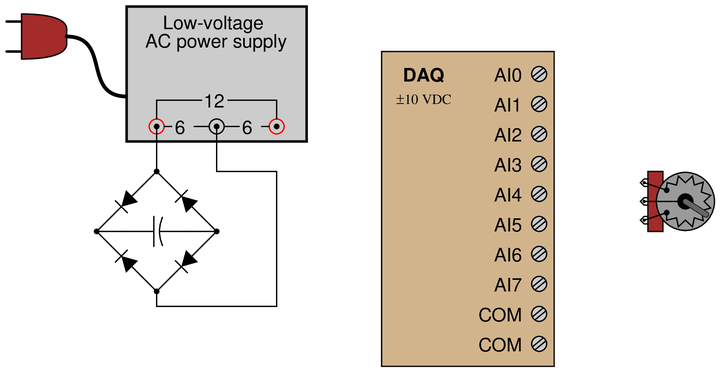• #### Question 17

Determine how to connect this DAQ unit to measure the output voltage of the Wheatstone bridge in such a way that an increasing compression on the strain gauge causes a {\it positive} indication at channel 3 of the DAQ, and that the same DAQ channel will register zero when the strain gauge is at rest: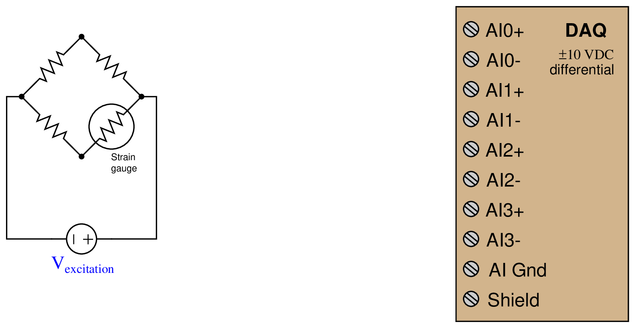• #### Question 18

Sketch connecting wires to allow this data acquisition unit (DAQ) to sense strain using quarter-bridge strain gauge circuits on input channels \#0 and \#3, such that increasing tension on the strain gauge (increasing gauge resistance) generates a more {\it positive} signal voltage on each channel: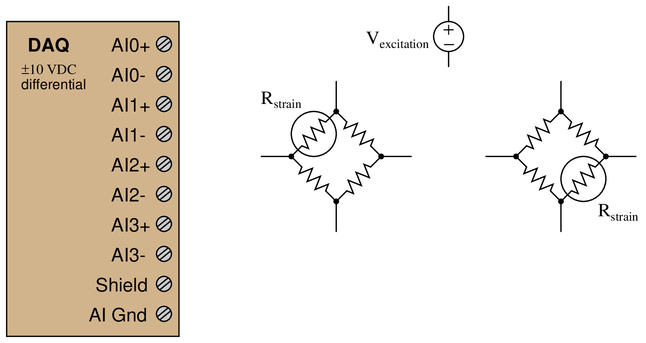• #### Question 19

Suppose we wished to use this DAQ unit to measure the {\it peak inverse voltage} across diode $D_3$ during operation of the power supply circuit. Identify how we should connect channel 1 of the DAQ to do this, assuming we want the DAQ to register a {\it positive} value at the moment in time of the diode’s peak inverse voltage: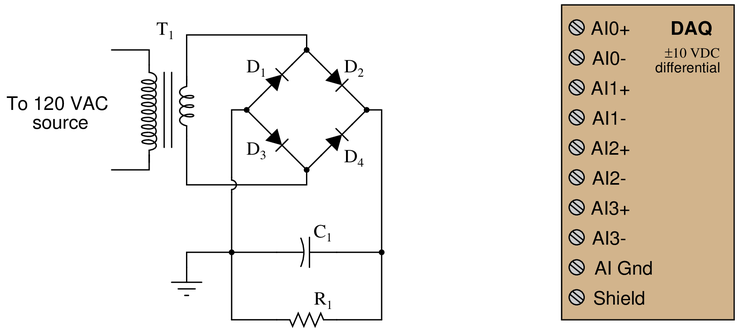• #### Question 20

Identify suitable input terminals, proper modes, and necessary connecting wires to allow this National Instruments E-series data acquisition unit (DAQ) to sense the two voltage sources shown: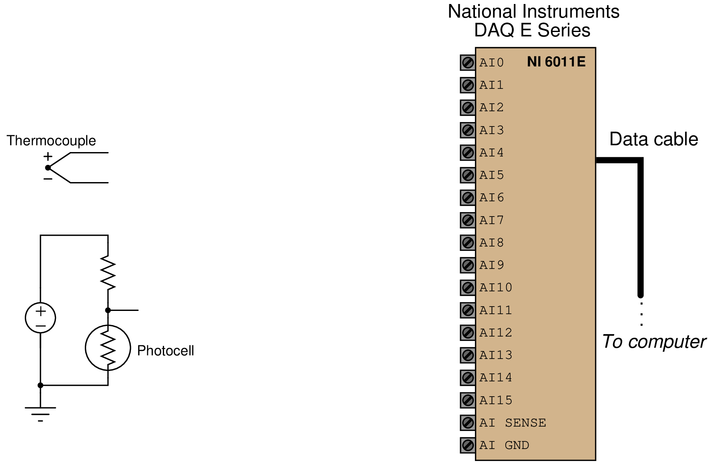The available modes for the input channels are RSE, NRSE, and DIFF:

$$\begin{array} {|l|l|} \hline Channel & Mode & First terminal & Second terminal \\ \hline 0 & & & \\ \hline 1 & & & \\ \hline \end{array}$$

• #### Question 21

Here, a temperature sensor called an {\it RTD} is used to translate ambient temperature into a proportional resistance. This in turn is converted into a proportional voltage signal by a constant current fed through the RTD by the current source: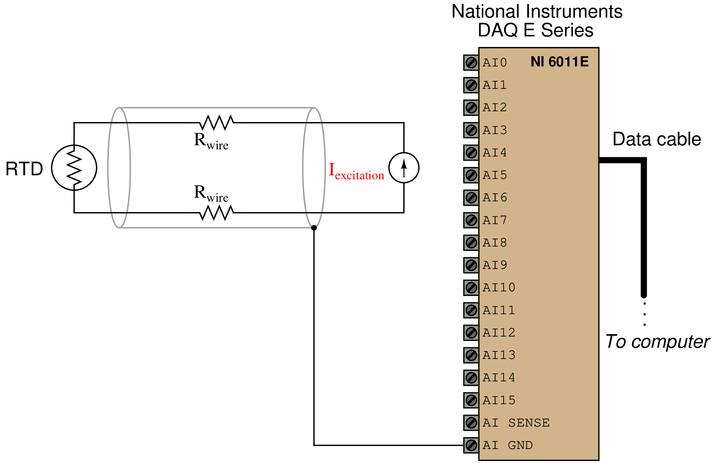Determine how to connect the first analog input channel this DAQ to measure the RTD’s voltage drop, but in such a way that voltage dropped along the cable’s length will not affect the measurement. Also, determine whether this DAQ should be configured for {\it single-ended} or {\it differential} input

• #### Question 22

Sketch a diagram showing all wire and tube connections between a primary sensing element, transmitter, controller, and/or final control element for an hypothetical control system. The instrument models will be randomly selected by your instructor, so that no two students will have the same combination of instruments. Your task is to locate the appropriate datasheets or manuals in the Instrumentation Reference (or online) to identify the proper wire terminals to connect, then sketch a simple loop diagram showing how the appropriate terminals on each device connect to terminals on the other devices to make a functional instrument loop. For instruments with multiple data channels, the instructor will also select which channel(s) to use for your loop (e.g. a PLC analog input card with 16 channels, the instructor may select channel \#5).

The instructor will assess the accuracy of your diagram when you present it along with documentation from the manufacturer showing how you identified each device’s terminals and connections. Fidelity to the manufacturer’s documentation is the only criterion for passing this mastery assessment.

The following lists recommend component types and models for the instructor to choose (randomly selecting one instrument from each list as applicable):

{\bf Primary Sensing Element / Switch options}

{\bullet} Discrete
item{\rightarrow} Mechanical limit switch with form-C contacts
item{\rightarrow} Mechanical process switch with form-C contacts
item{\rightarrow} Inductive proximity switch, sourcing
item{\rightarrow} Inductive proximity switch, sinking

{\bullet} Pressure
item{\rightarrow} Gauge pressure with single-block and bleed valves
item{\rightarrow} Gauge pressure with double-block and bleed valves
item{\rightarrow} Differential pressure with 3-valve manifold
item{\rightarrow} Differential pressure with 5-valve manifold

{\bullet} Level
item{\rightarrow} Rosemount 2120 vibrating fork level switch with relay output
item{\rightarrow} Rosemount 2120 vibrating fork level switch with PNP output

{\bullet} Temperature
item{\rightarrow} Thermocouple
item{\rightarrow} 2-wire RTD
item{\rightarrow} 3-wire RTD
item{\rightarrow} 4-wire RTD

{\bullet} Flow
item{\rightarrow} Orifice plate with isolation valves
item{\rightarrow} Turbine with pick-off coil

{\bullet} Analytical
item{\rightarrow} Glass pH probe
item{\rightarrow} Conductivity probe

{\bf Analog output transmitter options}

{\bullet} Pressure
item{\rightarrow} Rosemount 1151 Alphaline (analog)
item{\rightarrow} Rosemount 1151 HART (digital)
item{\rightarrow} Rosemount 3051 HART (digital)
item{\rightarrow} Yokogawa DPharp EJX110A
item{\rightarrow} Yokogawa DPharp EJX910
item{\rightarrow} Honeywell ST3000

{\bullet} Level

{\bullet} Temperature
item{\rightarrow} Rosemount 444
item{\rightarrow} Rosemount 644
item{\rightarrow} Rosemount 3044
item{\rightarrow} Rosemount 3144
item{\rightarrow} Foxboro RTT15
item{\rightarrow} Foxboro RTT30
item{\rightarrow} Moore Industries SPT with sourcing (4-wire) 4-20 mA output
item{\rightarrow} Moore Industries SPT with sinking (2-wire) 4-20 mA output
item{\rightarrow} Moore Industries TRX
item{\rightarrow} Moore Industries TDY

{\bullet} Flow
item{\rightarrow} Foxboro CFT50 coriolis

{\bullet} Analytical
item{\rightarrow} Rosemount 5081-P (pH)
item{\rightarrow} Daniel 700 gas chromatograph (4 analog output channels)
item{\rightarrow} Foxboro 876PH (pH/ORP/ISE)

{\bf Controller options -- single-loop and DCS}

{\bullet} Siemens 352P single-loop
{\bullet} Siemens 353 four-loop
{\bullet} Siemens 353R multi-loop
item{\rightarrow} Input module: IO-4TC (4-channel analog thermocouple)
item{\rightarrow} Input module: IO-4RT (4-channel analog RTD)
item{\rightarrow} Input module: IO-8AI-2 (8-channel analog current)
item{\rightarrow} Input module: IO-8AI-V (8-channel analog voltage)
item{\rightarrow} Input module: IO-8DI24DMN (8-channel discrete DC)
item{\rightarrow} Input module: IO-8DI24DSI (8-channel discrete DC)
item{\rightarrow} Input module: IO-8DI115AMN (8-channel discrete AC)
item{\rightarrow} Input module: IO-8DI115ASI (8-channel discrete AC)
item{\rightarrow} Output module: IO-8AO (8-channel analog current)
item{\rightarrow} Output module: IO-8DO60DMN (8-channel discrete DC)
item{\rightarrow} Output module: IO-8DO60DEI (8-channel discrete DC)

{\bullet} Foxboro 762CNA dual-loop
{\bullet} Foxboro 716C single-loop
{\bullet} Foxboro 718TC single-loop
{\bullet} Moore Industries 535 single-loop
{\bullet} Honeywell UDC2300 single-loop
{\bullet} Honeywell UDC3500 single-loop

item{\rightarrow} RTD input module
item{\rightarrow} AO analog output module

{\bullet} Emerson DeltaV DCS with M-series I/O cards
item{\rightarrow} Input module: M Series 2, DI 8-channel 24 VDC dry contact
item{\rightarrow} Input module: M Series 2, DI 8-channel 24 VDC isolated
item{\rightarrow} Input module: M Series 2, DI 8-channel 120 VAC dry contact
item{\rightarrow} Input module: M Series 2, DI 8-channel 120 VAC isolated
item{\rightarrow} Input module: M Series 2, AI 8-channel 4-20 mA
item{\rightarrow} Input module: M Series 2, AI 8-channel 1-5 VDC
item{\rightarrow} Input module: M Series 2, AI RTD, ohms
item{\rightarrow} Input module: M Series 2, AI thermocouple, mV
item{\rightarrow} Output module: M Series 2, DO 8-channel 24 VDC, high-side
item{\rightarrow} Output module: M Series 2, DO 8-channel 24 VDC, isolated
item{\rightarrow} Output module: M Series 2, DO 8-channel 120/230 VAC, high-side
item{\rightarrow} Output module: M Series 2, DO 8-channel 120/230 VAC, isolated
item{\rightarrow} Output module: M Series 2, AO 8-channel 4-20 mA

{\bullet} Honeywell Experion DCS with 2MLF-series I/O cards
item{\rightarrow} Input module: 2MLF-AC8A, 8-channel 4-20 mA
item{\rightarrow} Input module: 2MLF-AV8A, 8-channel 0-10 volt
item{\rightarrow} Input module: 2MLF-RD4A, 4-channel RTD
item{\rightarrow} Output module: 2MLF-DC4A, 4-channel 4-20 mA

{\bf Controller options -- PLC}

{\bullet} Siemens S7-300 I/O cards
item{\rightarrow} Input module: DI 32 x AC 120 V (6ES7321-1EL00-0AA0)
item{\rightarrow} Input module: DI 32 x DC 24 V (6ES7321-1BL00-0AA0)
item{\rightarrow} Input module: DI 16 x DC 24 V (6ES7321-1BH50-0AA0)
item{\rightarrow} Input module: DI 16 x DC 48-125 V (6ES7321-1CH20-0AA0)
item{\rightarrow} Input module: AI 8 x 16 bit (6ES7331-7NF00-0AB0)
item{\rightarrow} Output module: DO 32 x AC 120/230 V/1A (6ES7322-1FL00-0AA0)
item{\rightarrow} Output module: DO 16 x DC 24 V/0.5A (6ES7322-1BH01-0AA0)
item{\rightarrow} Output module: DO 16 x Rel (6ES7322-1HH01-0AA0)
item{\rightarrow} Output module: AO 8 x 12 bit (6ES7332-5HF00-0AB0)

{\bullet} Rockwell (Allen-Bradley) ControlLogix 5000 I/O cards
item{\rightarrow} Input module: 1756-IA16 (16-channel discrete 120 VAC)
item{\rightarrow} Input module: 1756-IB16 (16-channel discrete 24 VDC)
item{\rightarrow} Input module: 1756-IF6CIS (6-channel analog current)
item{\rightarrow} Input module: 1756-IF6I (6-channel analog isolated voltage/current)
item{\rightarrow} Input module: 1756-IF8 (8-channel analog differential voltage/current)
item{\rightarrow} Input module: 1756-IR6I (6-channel analog RTD)
item{\rightarrow} Input module: 1756-IT6I (6-channel analog thermocouple)
item{\rightarrow} Output module: 1756-OA8 (8-channel discrete 120 VAC)
item{\rightarrow} Output module: 1756-OB8 (8-channel discrete 24 VDC)
item{\rightarrow} Output module: 1756-OW16I (16-channel discrete relay)
item{\rightarrow} Output module: 1756-OF8 (8-channel analog voltage/current)
item{\rightarrow} Output module: 1756-OF6CI (8-channel analog current)

{\bf Final Control Element options}

{\bullet} Pneumatic control valve positioners
item{\rightarrow} Fisher 3582i positioner (4-20 mA input)
item{\rightarrow} Fisher DVC6000 positioner (4-20 mA input)

{\bullet} Electrically actuated valves (MOV)
item{\rightarrow} Limitorque actuator with Modutronic-20 II controller (4-20 mA input)
item{\rightarrow} Rotork AQ with Folomatic controller (4-20 mA input)

{\bullet} Electric solenoid valve (generic 24 VDC coil)
{\bullet} Electric solenoid valve (generic 120 VAC coil)

{\bullet} AC motor drives (VFD)
item{\rightarrow} Rockwell PowerFlex 4 (4-20 mA input)
item{\rightarrow} Rockwell PowerFlex 4 (discrete run’’ input in SNK mode)
item{\rightarrow} Rockwell PowerFlex 4 (discrete run’’ input in SRC mode)
item{\rightarrow} Automation Direct GS1 (4-20 mA input)
item{\rightarrow} Automation Direct GS1 (discrete forward’’ input)

• #### Question 23

Draw connecting wires that will create a {\it parallel} circuit, such that voltage will drop across each resistor in the polarity shown by the (+) and ($-$) symbols: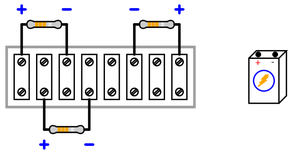{\bullet} Supposing the battery has a voltage of 1.5 volts, and all resistors are 1 k$\Omega$ in resistance value, calculate the voltage dropped by each resistor.
{\bullet} Supposing the battery has a voltage of 1.5 volts, and all resistors are 1 k$\Omega$ in resistance value, calculate the current passing through each resistor as well as the current passing through the battery.

• #### Question 24

Draw connecting wires that will create a {\it parallel} circuit with all the components shown: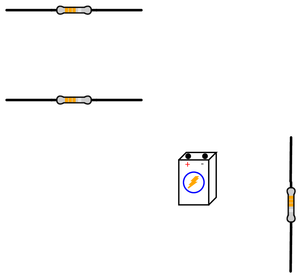{\bullet} Supposing the battery has a voltage of 6 volts, and all resistors are 1 k$\Omega$ in resistance value, calculate the voltage dropped by each resistor.
{\bullet} Supposing the battery has a voltage of 6 volts, and all resistors are 1 k$\Omega$ in resistance value, calculate the current passing through each resistor as well as the current passing through the battery.

• #### Question 25

Draw connecting wires that will create a {\it series} circuit, such that current (conventional flow notation) will follow the directions shown by the arrows near each resistor: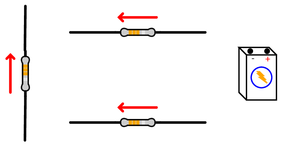{\bullet} Supposing the battery has a voltage of 12 volts, and all resistors are 1 k$\Omega$ in resistance value, calculate the voltage dropped by each resistor.
{\bullet} Supposing the battery has a voltage of 12 volts, and all resistors are 1 k$\Omega$ in resistance value, calculate the current passing through each resistor as well as the current passing through the battery.

• #### Question 26

Draw connecting wires that will create a {\it parallel} circuit, such that current (conventional flow notation) will follow the directions shown by the arrows near each resistor: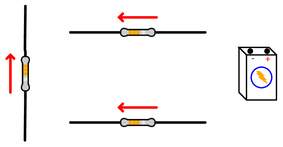{\bullet} Supposing the battery has a voltage of 3 volts, and all resistors are 1 k$\Omega$ in resistance value, calculate the voltage dropped by each resistor.
{\bullet} Supposing the battery has a voltage of 3 volts, and all resistors are 1 k$\Omega$ in resistance value, calculate the current passing through each resistor as well as the current passing through the battery.

• #### Question 27

Draw connecting wires that will create a {\it series} circuit, such that current (conventional flow notation) will follow the directions shown by the arrows near each resistor: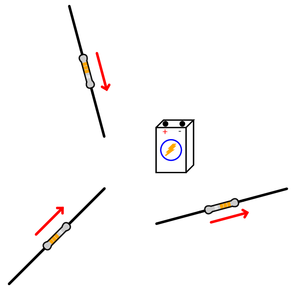{\bullet} Supposing the battery has a voltage of 4 volts, and all resistors are 1 k$\Omega$ in resistance value, calculate the voltage dropped by each resistor.
{\bullet} Supposing the battery has a voltage of 4 volts, and all resistors are 1 k$\Omega$ in resistance value, calculate the current passing through each resistor as well as the current passing through the battery.

• #### Question 28

Draw connecting wires that will create a {\it parallel} circuit, such that current (conventional flow notation) will follow the directions shown by the arrows near each resistor: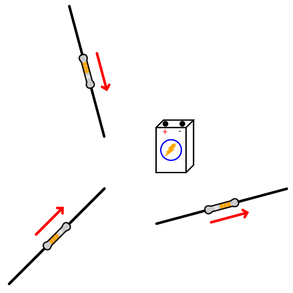{\bullet} Supposing the battery has a voltage of 8 volts, and all resistors are 1 k$\Omega$ in resistance value, calculate the voltage dropped by each resistor.
{\bullet} Supposing the battery has a voltage of 8 volts, and all resistors are 1 k$\Omega$ in resistance value, calculate the current passing through each resistor as well as the current passing through the battery.

• #### Question 29

Draw connecting wires that will create a {\it series} circuit with the three resistors and battery: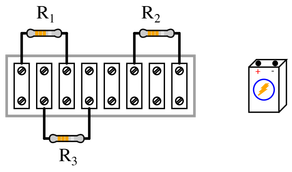{\bullet} Supposing the battery has a voltage of 15 volts, and all resistors are 1 k$\Omega$ in resistance value, calculate the voltage dropped by each resistor.
{\bullet} Supposing the battery has a voltage of 15 volts, and all resistors are 1 k$\Omega$ in resistance value, calculate the current passing through each resistor as well as the current passing through the battery.

• #### Question 30

Draw connecting wires that will create a {\it parallel} circuit with the three resistors and battery: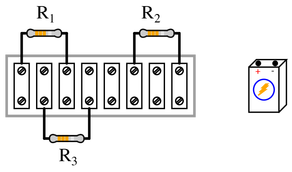{\bullet} Supposing the battery has a voltage of 12 volts, and all resistors are 1 k$\Omega$ in resistance value, calculate the voltage dropped by each resistor.
{\bullet} Supposing the battery has a voltage of 12 volts, and all resistors are 1 k$\Omega$ in resistance value, calculate the current passing through each resistor as well as the current passing through the battery.

• #### Question 31

Draw connecting wires that will create a {\it series} circuit, such that current (conventional flow notation) will follow the directions shown by the arrows near each resistor: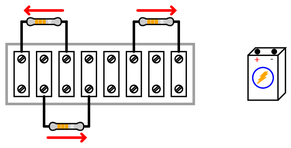{\bullet} Supposing the battery has a voltage of 10 volts, and all resistors are 1 k$\Omega$ in resistance value, calculate the voltage dropped by each resistor.
{\bullet} Supposing the battery has a voltage of 10 volts, and all resistors are 1 k$\Omega$ in resistance value, calculate the current passing through each resistor as well as the current passing through the battery.

• #### Question 32

Draw connecting wires that will create a {\it parallel} circuit, such that current (conventional flow notation) will follow the directions shown by the arrows near each resistor:{\bullet} Supposing the battery has a voltage of 7 volts, and all resistors are 1 k$\Omega$ in resistance value, calculate the voltage dropped by each resistor.
{\bullet} Supposing the battery has a voltage of 7 volts, and all resistors are 1 k$\Omega$ in resistance value, calculate the current passing through each resistor as well as the current passing through the battery.

• #### Question 33

Draw connecting wires that will create a {\it series} circuit, such that voltage will drop across each resistor in the polarity shown by the (+) and ($-$) symbols:{\bullet} Supposing the battery has a voltage of 1.5 volts, and all resistors are 1 k$\Omega$ in resistance value, calculate the voltage dropped by each resistor.
{\bullet} Supposing the battery has a voltage of 1.5 volts, and all resistors are 1 k$\Omega$ in resistance value, calculate the current passing through each resistor as well as the current passing through the battery.

• #### Question 34

Draw connecting wires that will create a {\it series} circuit with all the components shown:{\bullet} Supposing the battery has a voltage of 9 volts, and all resistors are 1 k$\Omega$ in resistance value, calculate the voltage dropped by each resistor.
{\bullet} Supposing the battery has a voltage of 9 volts, and all resistors are 1 k$\Omega$ in resistance value, calculate the current passing through each resistor as well as the current passing through the battery.

• #### Question 35

Resistors R_1 and R_2 are connected in parallel by virtue of being attached to the same two terminals on the terminal strip. Draw connecting wires that will create a {\it series} circuit between the parallel R_1/R_2 pair and the lone resistor R_3, such that voltage will drop across each resistor in the polarity shown by the (+) and ($-$) symbols: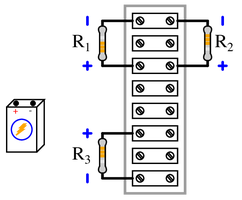{\bullet} Supposing the battery has a voltage of 11 volts, and all resistors are 1 k$\Omega$ in resistance value, calculate the voltage dropped by each resistor.
{\bullet} Supposing the battery has a voltage of 11 volts, and all resistors are 1 k$\Omega$ in resistance value, calculate the current passing through each resistor as well as the current passing through the battery.

• #### Question 36

Draw connecting wires that will create a {\it parallel} circuit between all three resistors, such that current (conventional flow notation) will go through each resistor as shown by the arrows: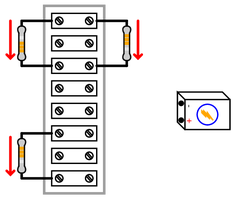{\bullet} Supposing the battery has a voltage of 14 volts, and all resistors are 1 k$\Omega$ in resistance value, calculate the voltage dropped by each resistor.
{\bullet} Supposing the battery has a voltage of 14 volts, and all resistors are 1 k$\Omega$ in resistance value, calculate the current passing through each resistor as well as the current passing through the battery.

• #### Question 37

Draw connecting wires that will create a {\it series} circuit between all three resistors, such that current (conventional flow notation) will go through each resistor as shown by the arrows: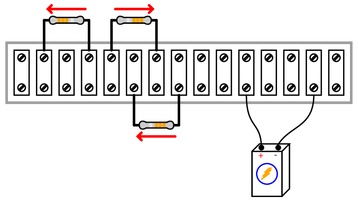{\bullet} Supposing the battery has a voltage of 18 volts, and all resistors are 1 k$\Omega$ in resistance value, calculate the voltage dropped by each resistor.
{\bullet} Supposing the battery has a voltage of 18 volts, and all resistors are 1 k$\Omega$ in resistance value, calculate the current passing through each resistor as well as the current passing through the battery.

• #### Question 38

Draw connecting wires that will create a {\it parallel} circuit, such that voltage will drop across each resistor in the polarity shown by the (+) and ($-$) symbols: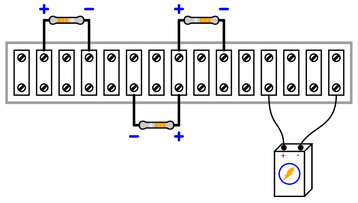{\bullet} Supposing the battery has a voltage of 2 volts, and all resistors are 1 k$\Omega$ in resistance value, calculate the voltage dropped by each resistor.
{\bullet} Supposing the battery has a voltage of 2 volts, and all resistors are 1 k$\Omega$ in resistance value, calculate the current passing through each resistor as well as the current passing through the battery.

• #### Question 39

Draw connecting wires that will create a {\it series-parallel} circuit, such that the voltage dropped across R_1 will be twice as much as the voltage dropped across R_2 or R_3. Make sure each resistor drops voltage in the polarity shown by the (+) and ($-$) symbols: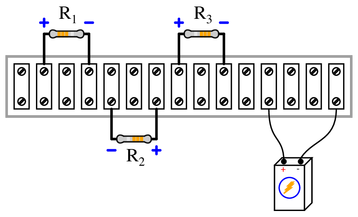{\bullet} Supposing the battery has a voltage of 12 volts, and all resistors are 1 k$\Omega$ in resistance value, calculate the voltage dropped by each resistor.
{\bullet} Supposing the battery has a voltage of 12 volts, and all resistors are 1 k$\Omega$ in resistance value, calculate the current passing through each resistor as well as the current passing through the battery.

• #### Question 40

Draw connecting wires that will create a {\it series-parallel} circuit, such that the voltage dropped across R_1 will be twice as much as the voltage dropped across R_2 or R_3. Make sure each resistor passes current (conventional flow notation) in the directions as shown by the arrows: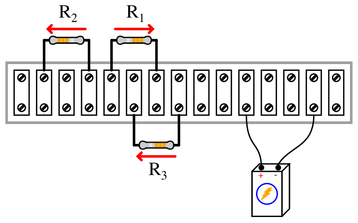{\bullet} Supposing the battery has a voltage of 18 volts, and all resistors are 1 k$\Omega$ in resistance value, calculate the voltage dropped by each resistor.
{\bullet} Supposing the battery has a voltage of 18 volts, and all resistors are 1 k$\Omega$ in resistance value, calculate the current passing through each resistor as well as the current passing through the battery.

• #### Question 41

Suppose we needed to connect a resistor in series with a sensitive analog meter movement to range that meter for a certain maximum voltage, and we were going to make all connections using a terminal strip. Draw connecting wires that will create a {\it series} circuit between the meter and the resistor, such that polarity of the applied voltage will be correct for the meter with the red test lead being positive and the black test lead being negative: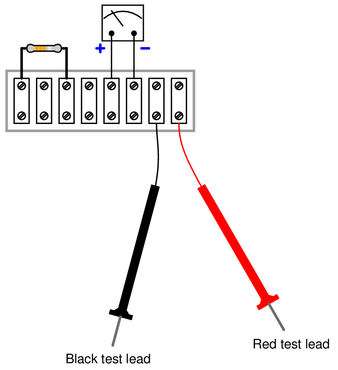• #### Question 42

Suppose we needed to connect a variable resistor in series with a sensitive analog meter movement to range that meter for a certain maximum voltage, and we were going to make all connections using a terminal strip. Draw connecting wires that will create a {\it series} circuit between the meter and two terminals of the potentiometer, such that polarity of the applied voltage will be correct for the meter with the red test lead being positive and the black test lead being negative: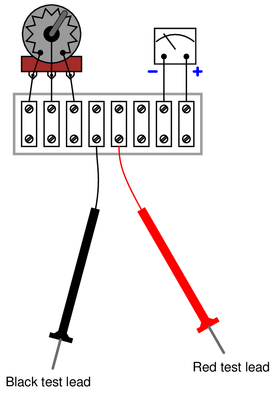• #### Question 43

Determine whether moving the potentiometer knob {\it clockwise} will increase or decrease the sensitivity of this analog voltmeter: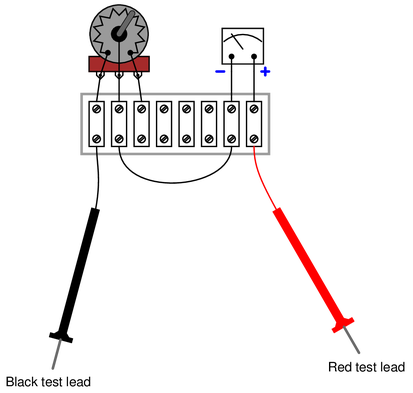{\bullet} Which way would we need to turn the potentiometer in order to make the voltmeter have a higher range (i.e. full-scale deflection represents a greater measured voltage value than before)